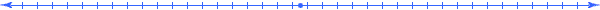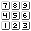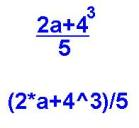###### How to Solve Word Problems Programmatically

Chapter

13Chapter 13.  Simplifying Expressions

 13.1 Substituting Numbers 13.2 Evaluating Numbers 13.3 Order of Operations 13.4 13.5 Using Rules to Simplify Expressions 13.6 Deriving Other Rules 13.7 Rules for Factoring 13.8 Rules for Exponents 13.9 Activities and Explorations

13.1

#### Substituting NumbersExpression Substitution Numbers Results (a+b)*c a=4, b=2, c=3 a*q2^q1 a=4, q1=2, q2=3 y/-x^2 y=8, x=2 third/first*second first=2, second=3, third=12 a+b*c a=4, b=2, c=3 q-r+s q=6, r=2, s=3 a+b+c a=2, b=4, c=5 5+4*x-3*x^2 x=2 a+x/b-x/c a=4, b=2, c=3, x=6 3*(x+2)-x^2 x=-3

Substitute the numbers into their corresponding expressions and calculate what you think is the value of the expression.  Do not worry about right or wrong.  The purpose of this exercise is to help you become aware of the conventions that mathematics uses to evaluate expressions.

Be aware that we use the sign "-" in two different ways, as a "negative" sign and as a "subtraction" operator.  The subtle difference between these two uses can cause problems if you do not take care.   One of the basic rules of algebra (discussed later) defines subtraction as adding the additive inverse (indicated by the negative sign) of a number:  a-b=a+(-b).

13.2

#### Evaluating NumbersExpression Substitution Numbers Results (a+b)*c a=4, b=2, c=3 (4+2)*3=6*3=18 a*q2^q1 a=4, q1=2, q2=3 4*(3^2)=36 or  (4*3)^2=144 y/-x^2 y=8, x=2 8/-(2)^2=-2 or 8/(-2)^2=4 third/first*second first=2, second=3, third=12 (12/2)*3=18 or 12/(2*3)=2 a+b*c a=4, b=2, c=3 2+(4*3)=14 or (2+4)*3=18 q-r+s q=6, r=2, s=3 (6-2)+3=7 or 6-(2+3)= 1 a+b+c a=2, b=4, c=5 (2+4)+5 = 2+(4+5)=11 5+4*x-3*x^2 x=2 multiple possibilities a+x/b-x/c a=4, b=2, c=3, x=6 multiple possibilities 3*(x+2)-x^2 x=-3 multiple possibilities

Some of these examples have more that one result that seem reasonable.  Shall we vote on them democratically?  Mathematics resolves this by a set of conventions (the language syntax) one of which specifies the "order of operations".  Try putting these examples into your calculator and make sure you know the proper keystrokes to get right answer as determined by the following order of operations.

13.3

#### Order of OperationsSign Operations Examples: (  ) paranthesis (a+b)*c (4+2)*3=6*3=18 ^ exponent a*q2^q1 4*3^2=36, not (4*3)^2=144 - negative y/-x^2 8/-(2)^2=-4, not 8/(-2)^2=4 / division third/first*second 12/2*3=18,  not 12/(2*3)=2 * multiplication a+b*c 2+4*3=14, not (2+4)*3=18 - subtraction q-r+s 6-2+3=7, not 6-(2+3)= 1 + addition a+b+c (2+4)+5 = 2+(4+5)

The order in which these operations appear in this table, determines which of these operators we do first.  Parenthesis always goes first; so when you want an expression to calculate first, put it in parenthesis.

 Traditional notation used in textbooks sometimes hides operations (especially multiplication, exponentiation and parenthesis).  By converting traditional notation to a text-string notation used by many computer software applications, we see explicit symbols for each operation and can determine more readily the sequence of operations calculated.These rules establish the conventions upon which we read a mathematical statement.  As demonstrated by the examples, understanding which operation we do first can make or break an effort to solve a word problem.  Now even the most complicated expressions end up with only one accepted value:

 Expression Substitution Numbers Results 5+4*x-3*x^2 x=2 5+4*x-3*x^2=5+8-12=1 a+x/b-x/c a=4, b=2, c=3, x=6 4+6/2-6/3=4+3-1=6 3*(x+2)-x^2 x=-3 3*(-3+2)-(-3)^2=-3-9=-12

13.4

#### Basic Algebra RulesRule Name Statement Example Simplify: (Addition) Identity a+0=a 2/3+3/2-6/4 Inverse a+(-a)=0 5+(1-6) Commutative a+b=b+a -4+5/2+4 Associative (a+b)+c=a+(b+c) (5+-6)+6 Definition of Subtraction a-b=a+(-b) 4-(-6) (Multiplication) Identity a*1 = a (4-8/4)*(3-2) Inverse a*(1/a)=1 6*8*(1/6) Commutative a*b=b*a 5*9/5 Associative (a*b)*c=a*(b*c) (3*4/5)*5 Definition of Division a/b=a*(1/b) 4/(1/4) (Connects Them) Distributive c*(a+b)=c*a+c*b 12(3/4+5/12)

These 11 rules form the foundation for algebra.  Just like geometry can be constructed from just 5 rules (Euclid's postulates), most of algebra can be derived from the above rules.  Go ahead and simplify each of the above examples to illustrate the corresponding rule (and sometimes use some of the other rules.)

13.5

#### Using Rules to Simplify ExpressionsRule Statement Example Results: (Addition) Identity a+0=a 3/4+3/2-6/4 3/4 Inverse a+(-a)=0 5+(1-6) 0 Commutative a+b=b+a -4+5/2+4 5/2 Associative (a+b)+c=a+(b+c) (5+-6)+6 5 Definition of Subtraction a-b=a+(-b) 4-(-6) 10 (Multiplication) Identity a*1 = a (4-8/4)*(3-2) 2 Inverse a*(1/a)=1 8*6*(1/6) 8 Commutative a*b=b*a 5*9*(1/5) 9 Associative (a*b)*c=a*(b*c) (3*4/5)*5 12 Definition of Division a/b=a*(1/b) 5*9/5 9 (Connects Them) Distributive c*(a+b)=c*a+c*b 12(3/4+5/12) 14

Did you get these results when you evaluated these expressions?   These rules allow us to simply expressions with variables just as easily as we evaluated these expressions.  To illustrate this, go ahead and use these basic rules to simplify the following expressions.

 Example Results: 3/4+6*x/2-3*x 5+(x-6*x)*(1/x) -4*x+5*x^2/2+4*x (5*x^2+-6*x)+6*x 4*x-(-6*x) (4*x-2)*(3*x+1-3*x) 8*x*(6+x)*(1/(6+x)) 5*x^3*9*(1/(5*x^3)) (3*x*4/5)*5 5*x^3*9/(5*x^3) 12(3*x/4+5*x/12)

Check out the results of simplifying these expressions that containing variables using (at least) the rule that it appears with.

 Rule Example Results: (Addition) Identity 3/4+6*x/2-3*x 3/4 Inverse 5+(x-6*x)*(1/x) 0 Commutative -4*x+5*x^2/2+4*x 5*x^2/2 Associative (5*x^2+-6*x)+6*x 5*x^2 Definition of Subtraction 4*x-(-6*x) 10*x (Multiplication) Identity (4*x-2)*(3*x+1-3*x) 4*x-2 Inverse 8*x*(6+x)*(1/(6+x)) 8*x Commutative 5*x^3*9*(1/(5*x^3)) 9 Associative (3*x*4/5)*5 12*x Definition of Division 5*x^3*9/(5*x^3) 9 Distributive 12(3*x/4+5*x/12) 14*x

13.6

#### Deriving Other Rules(a+b)^2=a^2+2*a*b+b^2   or equivalently   (a+b)2=a2+2*a*b+b2 Sum-Squared Rule

One nice feature of mathematics comes from the fact that if you cannot remember a formula (like this expansion formula), you can often remember related formulas and derive the forgotten one.  The 11 basic rules presented above form the foundation upon which we derive most of the other algebra rules.  Let us use them to derive (prove) the above sum-squared rule.

 Rule Reason (a+b)^2= (a+b)*(a+b)= Definition of exponent (a+b)*a+(a+b)*b= Distributive rule (from left) a*a+b*a+(a+b)*b= Distributive rule on left multiplication a*a+b*a+a*b+b*b= Distributive rule on right multiplication a*a+a*b+a*b+b*b= Commutative rule on second term a*a+2*a*b +b*b= Associative rule on two middle terms a^2+2*a*b +b^2 Definition of exponent

13.7

#### Rules for FactoringRule Example Result: (a+b)2=a2+2*a*b+b2 (5+x)^2 (a-b)2=a2-2*a*b+b2 (1-x)^2 a2-b2=(a+b)*(a-b) 1-x^2 (a*x+b)(c*x+d)=a*c*x2+(a*d+b*c)*x+b*d (2*x-3)*(5*x+4)

The last rule generalizes the factoring of a quadratic expression.  We often use it in reverse where we have the expression on the right of the equal sign and then determine the left side of the equation.  These techniques (usually covered in a second year algebra course) take some practice, but are helpful in simplifying fractions of expressions like the last added example.  The website http://icm.mcs.kent.edu/research/facdemo.html allows you to enter a polynomial and get factors, if they exist.  Try entering 10*x^2-7*x-12 and see what you get.

Did you get the following results for the above table of examples?

 Example Results: (5+x)^2 25+5*x+x^2 (1-x)^2 1-2*x+x^2 1-x^2 (1+x)(1-x) (2*x-3)*(5*x+4) 10*x^2-7*x-12 (10*x^2-7*x-12)/ (2*x-3) (5*x+4)

http://www-math.mit.edu/18.013A/tools/tools04.html  (Visual blocks to represent factors)

13.8

#### Rules for FractionsRule Example Result: (a/b)*b=a ((x+7)/4)*4 a/b+c/d=(a*d+c*b)/b*d x/2+y/6 a/b-c/d=(a*d-c*b)/b*d 1/3-2/A (a/b)*(c/d)=(a*c)/(b*d) (((x-5)^2)/5)*(5/(x-5)) (a/b)/(c/d)=(a/b)*(d/c) (4/c)/(c/2) (a/b)^n  = a^n / b^n (x/3)^n

Discussion of fraction rules can be found at

13.9

#### Rules for ExponentsRule Example Simplify: ax*ay=ax+y (2+y)5(2+y)-4 (ax)y=ax*y ((2 (1/x+1))2*x (a*b)x=ax*bx (1+x)3 * (2/(1+x))3 ax/ay=ax-y z5/z3 a-x=1/ax (2+y)4*(1/(2+y) 3) (a/b)x = ax/bx (1-x2)3/(1-x)3

13.10

#### Activities and ExplorationsActivities:

 Interactive Review of Some Algebra Rules   College Algebra Online Tutorial   Find Factors of Some Polynomials http://icm.mcs.kent.edu/research/facdemo.html

Explorations:

 Algebra As a Language   Review Fraction Rules http://library.thinkquest.org/20991/textonly/alg/frac.html

Exercises:

 Do Related Problems In Your Textbook

Copyright © 2004  Dr. Ranel E. Erickson